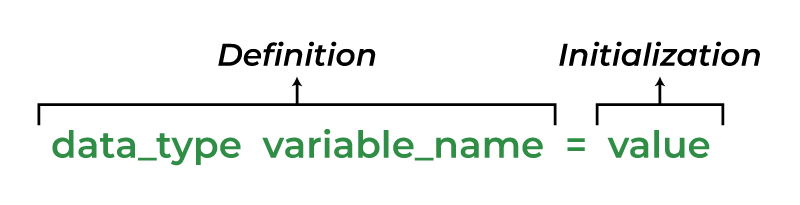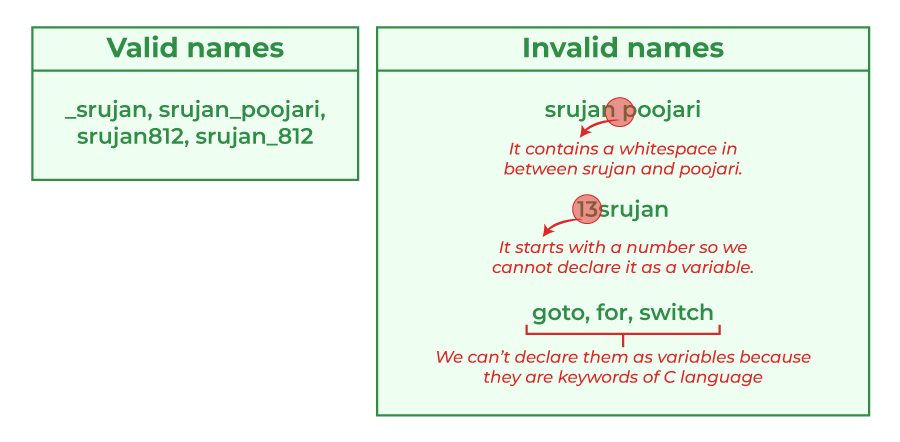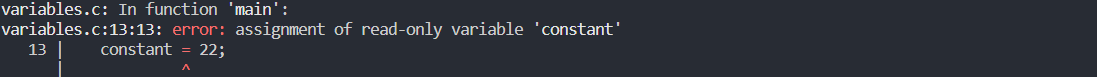Open In App
Related Articles
• Write an Interview Experience
• C Programming Language Tutorial

# C Variables

A variable in C language is the name associated with some memory location to store data of different types. There are many types of variables in C depending on the scope, storage class, lifetime, type of data they store, etc. A variable is the basic building block of a C program that can be used in expressions as a substitute in place of the value it stores.

## What is a variable in C?

A variable in C is a memory location with some name that helps store some form of data and retrieves it when required. We can store different types of data in the variable and reuse the same variable for storing some other data any number of times.

They can be viewed as the names given to the memory location so that we can refer to it without having to memorize the memory address. The size of the variable depends upon the data type it stores.

## C Variable Syntax

The syntax to declare a variable in C specifies the name and the type of the variable.

```data_type variable_name = value;    // defining single variable
or
data_type variable_name1, variable_name2;    // defining multiple variable```

Here,

• data_type: Type of data that a variable can store.
• variable_name: Name of the variable given by the user.
• value: value assigned to the variable by the user.

Example

```int var;    // integer variable
char a;     // character variable
float fff;  // float variables```

Note: C is a strongly typed language so all the variables types must be specified before using them.Variable Syntax Breakdown

There are 3 aspects of defining a variable:

1. Variable Declaration
2. Variable Definition
3. Variable Initialization

### 1. C Variable Declaration

Variable declaration in C tells the compiler about the existence of the variable with the given name and data type. No memory is allocated to a variable in the declaration.

### 2. C Variable Definition

In the definition of a C variable, the compiler allocates some memory and some value to it. A defined variable will contain some random garbage value till it is not initialized.

Example

```int var;
char var2;```

Note: Most of the modern C compilers declare and define the variable in single step. Although we can declare a variable in C by using extern keyword, it is not required in most of the cases. To know more about variable declaration and definition, click here.

### 3. C Variable Initialization

Initialization of a variable is the process where the user assigns some meaningful value to the variable.

Example

```int var; // variable definition
var = 10; // initialization
or
int var = 10; // variable declaration and definition```

## How to use variables in C?

The below example demonstrates how the use variables in C language.

## C

 `// C program to demonstrate the``// declaration, definition and``// initialization``#include ` `int` `main()``{``    ``// declaration with definition``    ``int` `defined_var;` `    ``printf``(``"Defined_var: %d\n"``, defined_var);` `    ``// initialization``    ``defined_var = 12;` `    ``// declaration + definition + initialization``    ``int` `ini_var = 25;` `    ``printf``(``"Value of defined_var after initialization: %d\n"``,defined_var);``    ``printf``(``"Value of ini_var: %d"``, ini_var);` `    ``return` `0;``}`

Output

```Defined_var: 0
Value of defined_var after initialization: 12
Value of ini_var: 25```

## Rules for Naming Variables in C

You can assign any name to the variable as long as it follows the following rules:

1. A variable name must only contain alphabets, digits, and underscore.
3. No whitespace is allowed within the variable name.
4. A variable name must not be any reserved word or keyword.## C Variable Types

The C variables can be classified into the following types:

1. Local Variables
2. Global Variables
3. Static Variables
4. Automatic Variables
5. Extern Variables
6. Register Variables

## 1. Local Variables in C

A Local variable in C is a variable that is declared inside a function or a block of code. Its scope is limited to the block or function in which it is declared.

## C

 `// C program to declare and print local variable inside a``// function.``#include ` `void` `function()``{``    ``int` `x = 10; ``// local variable``    ``printf``(``"%d"``, x);``}` `int` `main() { function(); }`

Output

`10`

In the above code, x can be used only in the scope of function(). Using it in the main function will give an error.

## 2. Global Variables in C

A Global variable in C is a variable that is declared outside the function or a block of code. Its scope is the whole program i.e. we can access the global variable anywhere in the C program after it is declared.

## C

 `// C program to demonstrate use of global variable``#include ` `int` `x = 20; ``// global variable` `void` `function1() { ``printf``(``"Function 1: %d\n"``, x); }` `void` `function2() { ``printf``(``"Function 2: %d\n"``, x); }` `int` `main()``{` `    ``function1();``    ``function2();``    ``return` `0;``}`

Output

```Function 1: 20
Function 2: 20```

In the above code, both functions can use the global variable as global variables are accessible by all the functions.

Note: When we have same name for local and global variable, local variable will be given preference over the global variable by the compiler.

For accessing global variable in this case, we can use the method mention here.

## 3. Static Variables in C

A static variable in C is a variable that is defined using the static keyword. It can be defined only once in a C program and its scope depends upon the region where it is declared (can be global or local).

The default value of static variables is zero.

### Syntax of Static Variable in C

`static data_type variable_name = initial_value;`

As its lifetime is till the end of the program, it can retain its value for multiple function calls as shown in the example.

## C

 `// C program to demonstrate use of static variable``#include ` `void` `function()``{``    ``int` `x = 20; ``// local variable``    ``static` `int` `y = 30; ``// static variable``    ``x = x + 10;``    ``y = y + 10;``    ``printf``(``"\tLocal: %d\n\tStatic: %d\n"``, x, y);``}` `int` `main()``{``    ``printf``(``"First Call\n"``);``    ``function();``    ``printf``(``"Second Call\n"``);``    ``function();``    ``printf``(``"Third Call\n"``);``    ``function();``    ``return` `0;``}`

Output

```First Call
Local: 30
Static: 40
Second Call
Local: 30
Static: 50
Third Call
Local: 30
Static: 60```

In the above example, we can see that the local variable will always print the same value whenever the function will be called whereas the static variable will print the incremented value in each function call.

Note: Storage Classes in C is the concept that helps us to determine the scope, lifetime, memory location, and default value (initial value) of a variable.

## 4. Automatic Variable in C

All the local variables are automatic variables by default. They are also known as auto variables.

Their scope is local and their lifetime is till the end of the block. If we need, we can use the auto keyword to define the auto variables.

The default value of the auto variables is a garbage value.

### Syntax of Auto Variable in C

```auto data_type variable_name;
or
data_type variable_name;    (in local scope)```

## C

 `// C program to demonstrate use of automatic variable``#include ` `void` `function()``{``    ``int` `x = 10; ``// local variable (also automatic)``    ``auto` `int` `y = 20; ``// automatic variable``    ``printf``(``"Auto Variable: %d"``, y);``}``int` `main()``{` `    ``function();``    ``return` `0;``}`

Output

`Auto Variable: 20`

In the above example, both x and y are automatic variables. The only difference is that variable y is explicitly declared with the auto keyword.

## 5. External Variables in C

External variables in C can be shared between multiple C files. We can declare an external variable using the extern keyword.

Their scope is global and they exist between multiple C files.

### Syntax of Extern Variables in C

`extern data_type variable_name;`

### Example of Extern Variable in C

```  ----------myfile.h------------
extern int x=10;  //external variable (also global)

----------program1.c----------
#include "myfile.h"
#include <stdio.h>
void printValue(){
printf("Global variable: %d", x);
}```

In the above example, x is an external variable that is used in multiple C files.

## 6. Register Variables in C

Register variables in C are those variables that are stored in the CPU register instead of the conventional storage place like RAM. Their scope is local and exists till the end of the block or a function.

These variables are declared using the register keyword.

The default value of register variables is a garbage value.

### Syntax of Register Variables in C

`register data_type variable_name = initial_value;`

## C

 `// C program to demonstrate the definition of register``// variable``#include ` `int` `main()``{``    ``//    register variable``    ``register` `int` `var = 22;` `    ``printf``(``"Value of Register Variable: %d\n"``, var);``    ``return` `0;``}`

Output

`Value of Register Variable: 22`

NOTE: We cannot get the address of the register variable using addressof (&) operator because they are stored in the CPU register. The compiler will throw an error if we try to get the address of register variable.

## Constant Variable in C

Till now we have only seen the variables whose values can be modified any number of times. But C language also provides us a way to make the value of a variable immutable. We can do that by defining the variable as constant.

A constant variable in C is a read-only variable whose value cannot be modified once it is defined. We can declare a constant variable using the const keyword.

### Syntax of Const Variable in C

`const data_type variable_name = value;`

Note: We have to always initialize the const variable at the definition as we cannot modify its value after defining.

## C

 `// C Program to Demonstrate constant variable``#include ` `int` `main()``{``    ``// variable``    ``int` `not_constant;` `    ``// constant variable;``    ``const` `int` `constant = 20;` `    ``// changing values``    ``not_constant = 40;``    ``constant = 22;` `    ``return` `0;``}`

Output## FAQs on C Variables

### Q1. What is the difference between variable declaration and definition in C?

Ans:

In variable declaration, only the name and type of the variable is specified but no memory is allocated to the variable.

In variable definition, the memory is also allocated to the declared variable.

### Q2. What is the variable’s scope?

Ans:

The scope of a variable is the region in which the variable exists and it is valid to perform operations on it. Beyond the scope of the variable, we cannot access it and it is said to be out of scope.